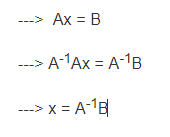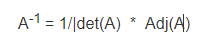Related Articles

# Inverse of Matrix in R

• Difficulty Level : Expert
• Last Updated : 22 Apr, 2020

The inverse of a matrix is just a reciprocal of the matrix as we do in normal arithmetic for a single number which is used to solve the equations to find the value of unknown variables. The inverse of a matrix is that matrix which when multiplied with the original matrix will give as an identity matrix.

Finding the inverse of a matrix is one of the most common tasks while working with linear algebraic expressions. We can find the inverse of only those matrices which are square and whose determinant is non-zero.

Note: Ensure that the matrix is non-singular that is the determinant should not be 0.

Matrix Equation:where,
A^-1 is the inverse of matrix A.
x is the unknown variable column.
B is the solution matrix.

Equation for Inverse of Matrix:There are two ways in which the inverse of a Matrix can be found:

• Using the solve() function:
`solve()` is a generic built-in function in R which is helpful for solving the following linear algebraic equation just as shown above in the image. It can be applied both on vectors as well as a matrix.
 `# R program to find inverse of a Matrix`` ` `# Create 3 different vectors``# using combine method.``a1 <``-` `c(``3``, ``2``, ``5``)``a2 <``-` `c(``2``, ``3``, ``2``)``a3 <``-` `c(``5``, ``2``, ``4``)`` ` `# bind the three vectors into a matrix ``# using rbind() which is basically``# row-wise binding.``A <``-` `rbind(a1, a2, a3)`` ` `# print the original matrix``print``(A)`` ` `# Use the solve() function ``# to calculate the inverse.``T1 <``-` `solve(A)`` ` `# print the inverse of the matrix.``print``(T1)`

Output:

```   [,1] [,2] [,3]
a1    3    2    5
a2    2    3    2
a3    5    2    4

a1          a2         a3
[1,] -0.29629630 -0.07407407  0.4074074
[2,] -0.07407407  0.48148148 -0.1481481
[3,]  0.40740741 -0.14814815 -0.1851852

```
• Using the inv() function:
`inv()` function is a built-in function in R which is especially used to find the inverse of a matrix.

Note:Ensure that you have installed the ‘matlib’ package in your environment.

Finding Determinant of Matrix:

 `# Create 3 different vectors.``a1 <``-` `c(``3``, ``2``, ``8``)``a2 <``-` `c(``6``, ``3``, ``2``)``a3 <``-` `c(``5``, ``2``, ``4``)`` ` `# Bind the 3 matrices row-wise ``# using the rbind() function.``A <``-` `rbind(a1, a2, a3)`` ` `# determinant of matrix``print``(det(A))`

Output:

`-28`

Finding Inverse of Matrix:

 `# find inverse using the inv() function.``print``(inv(t(A))) `

Output:

```
[1,] -0.2857143  0.5  0.1071429
[2,] -0.2857143  1.0 -0.1428571
[3,]  0.7142857 -1.5  0.1071429
```

My Personal Notes arrow_drop_up